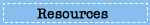# Sample Questions

## What questions are used in the NAEP mathematics assessment?

Explore sample questions from the mathematics assessment, and see how the NAEP mathematics questions relate to student performance.## Test Yourself in Mathematics

Select one of the grade tabs below to try out 10 questions from the 2009 NAEP mathematics assessment. After answering all the questions, compare your score with that of students nationally.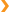Grade 12 Sample Questions

Question 1 of 10: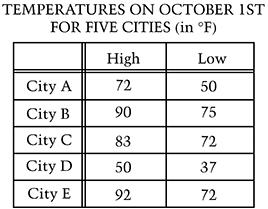The table above shows the high and low temperatures on October 1st for five cities. Which city had the greatest temperature range?

1. City A
2. City B
3. City C
4. City D
5. City E

Question 2 of 10:

John is going to cover an attic floor with insulation. The floor measures 25 feet by 35 feet. If one roll of insulation will cover 64 square feet, how many rolls of insulation does John need?

1. 1
2. 2
3. 8
4. 14
5. 110

Question 3 of 10:

The first four terms in a sequence are shown below.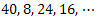Each term after the first two terms is found by taking one-half the sum of the two preceding terms. Which term is the first odd number in this sequence?

1. The 5th term
2. The 6th term
3. The 7th term
4. The 8th term
5. The 9th term

Question 4 of 10: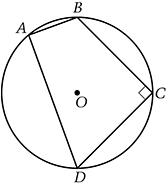Quadrilateral ABCD is inscribed in circle O , and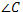is a right angle, as shown above. Segment AB is not parallel to segment DC . Which of the following statements must be true?

1.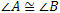2.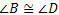3.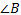is a right angle.
4.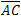is a diameter of circle O.
5.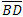is a diameter of circle O.

Question 5 of 10:

The manager of a company has to order new engines for its delivery trucks after the trucks have been driven 150,000 miles. One of the delivery trucks currently has 119,866 miles on it. This truck has the same delivery route each week and is driven an average of 40,000 miles each year. At this rate, the manager should expect this truck to reach 150,000 miles in approximately how many months?

1. Less than 4 months
2. Between 4 and 6 months
3. Between 6 and 8 months
4. Between 8 and 10 months
5. More than 10 months

Question 6 of 10:

Which of the following expressions is NOT equivalent to (a + b) (x + y) ?

1. (a + b) x + (a + b) y
2. a (x + y) + b (x + y)
3. (b + a) (y + x)
4. ax + by
5. ax + bx + ay + by

Question 7 of 10:

The principal of a high school would like to determine why there has been a large decline during the year in the number of students who buy food in the school’s cafeteria. To do this, 25 students from the school will be surveyed. Which method would be the most appropriate for selecting the 25 students to participate in the survey?

1. Randomly select 25 students from the senior class.
2. Randomly select 25 students from those taking physics.
3. Randomly select 25 students from a list of all students at the school.
4. Randomly select 25 students from a list of students who eat in the cafeteria.
5. Give the survey to the first 25 students to arrive at school in the morning.

Question 8 of 10: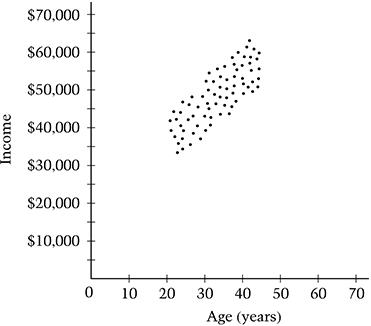A random sample of graduates from a particular college program reported their ages and incomes in response to a survey. Each point on the scatterplot above represents the age and income of a different graduate.

Of the following equations, which best fits the data above?

1. y = - 1,000x + 15,000
2. y = - 1,000x
3. y = 1,000x + 15,000
4. y = 10,000x
5. y = 10,000x + 15,000

Question 9 of 10:

Angie has a bag containing n apples. She gives 4 to her brother and keeps 5 for herself. She then divides the remaining apples equally among 3 friends. Which of the following expressions represents the number of apples each friend receives?

1.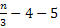2.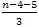3.4.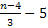5.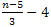Question 10 of 10:

Carlene told Kyle that a rectangular room measured 16 feet by 12 feet, to the nearest foot. This means that the length could measure between 15.5 feet and 16.5 feet and the width could measure between 11.5 feet and 12.5 feet.

Kyle performed the following calculations.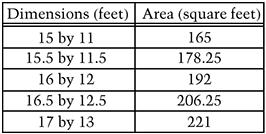Of the following intervals, which is the smallest interval that contains all possible values of the area of the room?

1. Between 191.5 and 192.5 square feet
2. Between 191 and 193 square feet
3. Between 179 and 206 square feet
4. Between 178 and 207 square feet
5. Between 165 and 221 square feetThe chart below shows the percentage of questions that you answered correctly, as well as how students at each of the achievement levels performed on this set of questions. For instance, twelfth-grade students at Proficient had a percentage correct of 80% on average.
•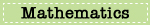•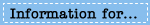•